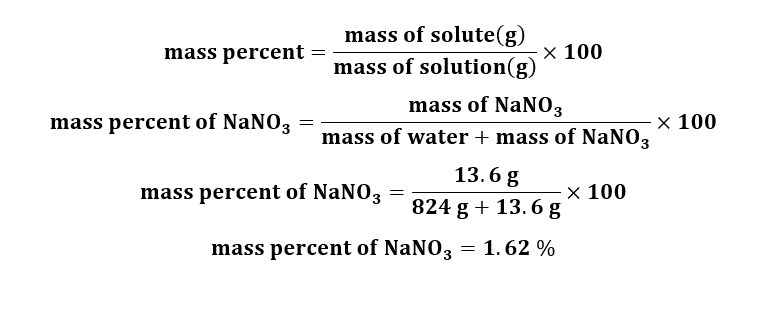# (a) Calculate the mass percentage of NaNO3 in a solution containing 13.6 g of NaNO3 in 834 g of water. (b) An alloy contains 2.86 g of chromium per 100 kg of alloy. What is the concentration of chromium in ppm?

Question
10 views

(a) Calculate the mass percentage of NaNO3 in a solution containing 13.6 g of NaNO3 in 834 g of water. (b) An alloy contains 2.86 g of chromium per 100 kg of alloy. What is the concentration of chromium in ppm?

check_circle

(a) Calculation for the mass percentage of NaNO3 in a solution:

Given:

Mass of NaNO3 = 13.6 g

Mass of water (H2O) = 824 g

Calculation for mass percent of NaNO3:Anwer:

The mass percent of NaNO3 in solution is 1.62%.

...

### Want to see the full answer?

See Solution

#### Want to see this answer and more?

Solutions are written by subject experts who are available 24/7. Questions are typically answered within 1 hour.*

See Solution
*Response times may vary by subject and question.
Tagged in

### Chemistry